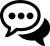# PowerShell Loops## Daily PowerShell #10

####Discuss this Article

One of the basic features of any programming language is the loop structure. In this blog post, we will look at the basics of loops.

## For Loops

A `for` loop is controlled by an iterator variable and condition. Iterate loops are good for looping an exact number of times or for accessing elements of an array by index.

### Basic For Loop

A basic `for` loop can be found below. It is broken into three sections. The first section defines the the starting iterator value. In this case `\$i = 0` ensures that the iterator starts at zero. The second section, separated by a semicolon, defines the condition at which the loop will stop iterating. In this case, `\$i -lt 5` ensures the iteration continues until `\$i` greater than or equal to 5. Finally, the third section defines the increment per iteration. In this case, the `\$i++` increments by one for each loop.

``````for(\$i = 0; \$i -lt 5; \$i++)
{
\$i
}

<# Output
0
1
2
3
4
#>
``````

### Reverse For Loop

Instead of looping forward, you can loop backwards by decrementing for each iteration.

``````for(\$i = 5; \$i -gt 0; \$i--)
{
\$i
}

<# Output
5
4
3
2
1
#>
``````

### For Loop Over an Array

For loops can be used to iterate arrays by index. Arrays are zero indexed so you want to start the iterator at zero and loop the length of the array.

``````\$Array = @(2, 9, 3, 4, 0)
for(\$i = 0; \$i -lt \$Array.Length; \$i++)
{
\$Array[\$i]
}

<# Output
2
9
3
4
0
#>
``````

### Storing the Output of a For Loop

You can store the output of a for loop by including a variable assignment on the left hand side of the for statement.

``````\$Items = for(\$i = 5; \$i -gt 0; \$i--)
{
\$i
}
<# Output

\$Items = @(5,4,3,2,1)

#>
``````

### Break Out of a For Loop

You can use the `break` keyword to stop iterating during a for loop.

``````for(\$i = 5; \$i -gt 0; \$i--)
{
if (\$i -eq 3)
{
break
}
\$i
}
<#
Output
5
4
#>
``````

When using loops, you need to use multiple break statements. The `break` statement will only break out of the inner most loop.

``````for(\$i = 5; \$i -gt 0; \$i--)
{
for(\$j = 5; \$j -gt 0; \$j--)
{
if (\$j -eq 3)
{
break
}
"J: \$j"
}

if (\$i -eq 3)
{
break
}

"I: \$i"
}
<#
Output
J: 5
J: 4
I: 5
J: 5
J: 4
I: 4
J: 5
J: 4
#>
``````

### Continue to the Next Iteration

You can use the `continue` statement to skip to the next iteration without finishing the execution of the body of the loop.

``````for(\$i = 5; \$i -gt 0; \$i--)
{
if (\$i -eq 3)
{
continue
}
\$i
}

<# Output
5
4
2
1
#>
``````

## For Each Loop

The `foreach` loop can be used to iterate over each item in an array without need to control the index values.

### Basic Foreach Loop

A basic `foreach` loop is shown below. The foreach loop will iterate over each item in the array in order.

``````\$Array = @(1, 2, 3, 4, 5)
foreach(\$item in \$array)
{
\$item
}
<# Output
1
2
3
4
5
#>
``````

### Breaking out of a Foreach Loop

Similar to a for loop, you can break out of a foreach loop with a `break` statement.

``````\$Array = @(1, 2, 3, 4, 5)
foreach(\$item in \$array)
{
if (\$item -eq 3)
{
break
}
\$item
}
<# Output
1
2
#>
``````

### Continue to the Next Iteration

Similar to a for loop, you can continue to the next iteration with the `continue` statement.

``````\$Array = @(1, 2, 3, 4, 5)
foreach(\$item in \$array)
{
if (\$item -eq 3)
{
continue
}
\$item
}
<# Output
1
2
4
5
#>
``````

### Store the result of a foreach loop in a variable

You can store the result of a `foreach` loop in a variable by including a variable assignment on the left hand side of the loop.

``````\$Array = @(1, 2, 3, 4, 5)
\$Items = foreach(\$item in \$array)
{
\$item
}
<# Output
\$Items = @(1,2,3,4,5)
#>
``````

## While Loop

The while loop does not control the iteration via items or an iterator variable. You need to provide a condition to continue with the loop.

### Basic While Loop

The following while loop checks `\$x` to make sure it’s less than.

``````\$x = 0
while(\$x -lt 5) {
\$x++
\$x
}
<# Output
1
2
3
4
5
#>
``````

### Do While Loop

A do while loop is a variation of a while loop that ensures that the loop executes at least once before checking the condition. Although `\$x` is less than 5, it will execute once before exiting.

``````\$x = 0
do {
\$x
} while (\$x -gt 5)
<# Output
0
#>
``````

### Looping Forever

You can create a loop that executes forever by placing `\$true` in the while condition. Press `Ctrl+C` to terminate the loop.

``````while(\$true) {
Start-Sleep 1
Get-Date
}
``````